# Dynamic Stability Analysis of Functionally Graded Timoshenko Beams with Internal Viscous Damping Distribution

Dynamic Stability Analysis of Functionally Graded Timoshenko Beams with Internal Viscous Damping Distribution

Mohamed BouamamaAbbes Elmeiche Abdelhak Elhennani Tayeb Kebir

Laboratory Mechanics of Structures and Solids, Department of Mechanical Engineering, Faculty of Technology, University of Sidi Bel Abbes 22000, Algeria

Laboratory of Materials and Systems Reactive, Department of Mechanical Engineering, Faculty of Technology, University of Sidi Bel Abbes, Algeria

Corresponding Author Email:
bouamamamohameddoc@gmail.com
Page:
341-346
|
DOI:
https://doi.org/10.18280/jesa.520402
Received:
2 April 2019
|
Accepted:
27 June 2019
|
Published:
10 October 2019
| Citation

OPEN ACCESS

Abstract:

This paper investigates the dynamic stability of functionally graded material (FGM) beams, using the Timoshenko model with internal viscous damping distribution (DIVD). It is assumed that the material properties change continuously across the thickness direction of the beam, according to the power law function for the FGM (P-FGM). The governing equations of motion were developed by the Lagrange principle. Then, the finite element method was adopted to describe unamortized natural frequencies and the damped eigenfrequencies of the dynamic system. The numerical results were compared with those available in the literature. Illustrative examples were given to show the influence of internal damping distribution, material distribution and slenderness ratio on the dynamic stability margin of FGM Timoshenko beams. The research findings provide new insights into the dynamic stability of the FGM.

Keywords:

dynamic stability, functionally graded material (FGM), Timoshenko beam, internal viscous damping, finite element method, eigenfrequencies

1. Introduction

Nowadays elastic components such as machines and structures have found a place in different industrial applications.  However, those types of elements are subjected to different type of dynamic motions and vibrations, which are mostly undesirable and can lead to a catastrophic failure. Therefore the need to measure and detect the vibration frequencies is crucial task to be able design a proper damping system.

Damping has a very high importance in structures subject to dynamic loading. Damping treatment is one of the methods for controlling noise and vibration in structures . The dissipation of energy depends on a large number of factors [2-4], internal factors (type of material, chemical composition, crystalline or amorphous structure), external factors (temperature, initial load, initial stress, etc.) and factors related to the movement (amplitude and frequency of deformation, state of the effort, etc.). For the study of damping influence different mathematical models exist (for example model of Newton, temporal hysteresis, fractional derivatives, Kelvin-Voigt, Maxwell and others).

The beam is considered as the structural element most responded, as an integral part in most of the construction works or machinery parts , which made necessary the study of their static / dynamic behavior. The beams are used as structural component in several construction applications and a large number of studies can be found in the literature about the transverse vibration of the uniform isotropic beams . The importance of developing an analysis of the vibration behavior of the beams is related to the use of the beams as a basic element in achieving the structures, and to determine the effects of changes in boundary conditions, and changes of the material on the natural frequencies and natural modes of vibration.

Recently, the dynamic behavior of structural elements in FGM is of considerable importance in the fields of research and industry. Typically these materials are made from a mixture of metal and ceramic, or a combination of materials. The ceramic component provides a high temperature because of its low resistance to thermal conductivity. FGMs are used in very different engineering applications such as automotive, aerospace, defense, and more recently electronics, nuclear reactors, biomedical and transportation.

Several studies have been conducted on the mechanical and thermal behavior of FGM [7-11]. The simplest of the FGM, two different materials gradually change from one to the other  Aydogdu and Taskin  studied the free vibration of functional graded beams with a simply supported boundary condition, Bouamama et al.  studied the frequency response of the FGM sandwich beam with different boundary conditions and volume fractions, Alshorbagy et al.  studied the characteristics of Euler-Bernoulli beams for the free vibration of FGM beams both axially and transversely across the beam thickness.

Other teams are interested in the static and stability analysis of nanobeams in FGM [15-18].

The effects of generalized damping distributions on non-homogeneous Timoshenko beams were investigated by . Dohnal et al.  studied the damping of a Cantilever beam under a periodic axial force by a finite-element approach. The effects of internal and/or external lumped or distributed damping have been extensively investigated by Robu, et al., Chang, et al, Lin, et al., Chen, and Bendine, et al. [21-25]. Capsoni et al.  who proposed a damper design technique absorber based on modal control for controlling the vibrations of beams. Van de Vegte and de Silva  Are studied the dynamic response of the aluminum beam with viscous internal damping using the Kelvin-Voigt material, Tsai, et al.  studied the effects of internal damping, the length and location of the damped segment on the vibration of the beam.

Our present study aims at finite element modeling to analyze the dynamic stability of a Timoshenko FGM beam in by the inclusion of viscous internal damping.

2. Functionnally Graded Beams Modelisation

Consider a Functionally Graded beams of length L and uniform thickness h, see Figure 1. The upper and lower faces of the beam are at $\mathrm{Z}=\pm \frac{\mathrm{h}}{2}$. The Functionally Graded Material (FGM) vary continuously due to the gradual change in the volume fraction of the constituent materials (ceramic and metal), generally in the thickness direction. The power law function P-FGM is commonly used to describe these variations properties of the materials.

## 1.png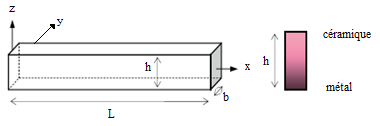Figure 1. FGM beams modeling

## 2.pngFigure 2. Variation of the Young's modulus in P-FGM beams

The volume fraction of the P-FGM beam is assumed to vary according to the thickness of the beam according to a simple power law function :

$g(z)=\left(\frac{z+\frac{h}{2}}{h}\right)^{p}$ (1)

where, g(z) denotes the function of the volume fraction of the beam; (p) is the power index who writes the variation profile of the materials according to the FGM beam thickness. The effective material properties can be expressed by a mixing law :

$\mathrm{P}(z)=\left(\mathrm{P}_{1}-P_{2}\right) g(z)+P_{2}$ (2)

wherein, P2 and P1 are respectively the material properties, such as Young’s modulus E, Poisson’s ratio v, and the mass density $\rho$, of the bottom surface (z = -h/2) and the top surface (z = h / 2) of the FGM beams.

It is clear that the mechanical properties of the P-FGM beam; Young's modulus for example (see Figure 2), decreases rapidly near the bottom surface for "p> 1", and increases rapidly near the top surface for "p <1". When "p = 1", the variation of Young's modulus becomes linear.

3. Theoretical Formulations

The dynamic stability analysis of FGM beams is based on Kelvin-Voigt's model, which expresses the mechanical behavior of a viscous solid. This model can be represented by a purely viscous damper and a parallel Hookean spring as shown in the (Figure 3).

## 3.png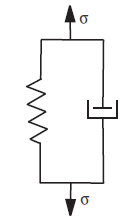Figure 3. Kelvin-Voigt model

The chosen damping model produces deceleration forces resulting from the damping effects during movement. The internal viscous damping distribution (DIVD) is derived from the damping of Kelvin-Voigt deformation velocity, where shear and additional dissipative bending stresses $\left\{\sigma^{\mathrm{d}}\right\}$ are linearly proportional to the strain rate by the coefficient β. The constraint is related to the rate of strain is given by the relation:

$\left\{\sigma^{T}\right\}=\left\{\sigma^{c}\right\}+\left\{\sigma^{d}\right\}=[Q] \cdot[\{\varepsilon\}+\beta \cdot\{\dot{\varepsilon}\}]$ (3)

4. Governing Equation

Assuming that Timoshenko's kinematic theory on FGM beams with distributed internal viscous damping (DIVD). The governing equations of motion can be obtained by applying the Hamilton principle to the Lagrangian $\mathscr{L}$ and the Rayleigh dissipation function $\mathscr{L}$ into the dynamic problem.

$\delta \int_{t_{0}}^{t_{1}}(\mathscr{Q}-\mathcal{R}) \cdot d t=\delta \int_{t_{0}}^{t_{1}}(T-V-\mathcal{R}) \cdot d t=0$ (4)

where, T and V denote the strain energy and kinetic energy of a functionally graded beam, respectively. The Expression of the kinetic energy T is given by the equation:

$\mathrm{T}=\frac{1}{2} \int_{\mathrm{V}} \rho(\mathrm{z}) \cdot\left(\mathrm{U}^{2}+\dot{\mathrm{W}}^{2}\right) \cdot \mathrm{d} \mathrm{V}$ (5)

$\dot{\mathrm{U}}$ and $\dot{\mathrm{W}}$ are the general speed components of the FGM beams respectively, along the (x) and (z) axes. $\rho(z)$ is The mass per unit volume.

The kinetic energy becomes in the form:

$\mathrm{T}=\frac{1}{2} \int_{0}^{\mathrm{L}}\left[\mathrm{I}_{1} \dot{\mathrm{u}}^{2}+\mathrm{I}_{2} \dot{\theta}^{2}\right] \cdot \mathrm{d} \mathrm{x}$ (6)

where,

$\left(I_{1}, I_{2}\right)=\int_{A} \rho(z) \cdot\left(1, z^{2}\right) \cdot d A$ (7)

The Total Potential Energy is defined as:

$V^{T}=\int_{v} \sigma_{i j}^{T} \cdot \varepsilon_{i j} d v=\int_{v} \sigma_{i j}^{c} \cdot \varepsilon_{i j} d v+\int_{v} \sigma_{i j}^{d} \cdot \varepsilon_{i j} d v$ (8)

$V^{T}=V+\mathcal{R}$ (9)

With:

$V=\int_{v} \sigma_{i j}^{c} \cdot \varepsilon_{i j} d v$

$\mathcal{R}=\int_{v} \sigma_{i j}^{d} \cdot \varepsilon_{i j} d v$

The effective Potential Energy can be calculated by:

$V=\frac{1}{2} \int_{0}^{L}\left[D_{11} \theta^{\prime 2}+\kappa A_{55}\left(u^{\prime}-\theta\right)^{2}\right] d x$ (10)

where,

$D_{11}=b \cdot \int_{-\frac{h}{2}}^{+\frac{h}{2}} \frac{E(z) \cdot z^{2}}{\left(1-v(z)^{2}\right)} \cdot d z$

$A_{55}=b \cdot \int_{-\frac{h}{2}}^{+\frac{h}{2}} \frac{E(z) \cdot z^{2}}{(1+v(z))} \cdot d z$

The Lagrangian $\mathscr{Q}$ could be written in the following form:

$\mathscr{Q}=\frac{1}{2} \int_{0}^{L}\left\{\mathrm{I}_{1} \dot{\mathrm{u}}^{2}+I_{2} \dot{\theta}^{2}-D_{11}\left[\theta^{\prime 2}+\frac{A_{55} \kappa}{D_{11}}\left(\mathrm{u}^{\prime}-\theta^{\prime}\right)^{2}\right]\right\} d x$ (11)

The Rayleigh dissipation function $\mathcal{R}$ can be obtained as follows:

$\mathcal{R}=\frac{1}{2} \int_{0}^{L}\left[\beta \kappa D_{1 l} \dot{\theta}^{2}+\beta \kappa A_{s 5}\left(\dot{\mathfrak{u}}^{\prime}-\dot{\theta}^{\prime}\right)^{2}\right] d x$ (12)

5. Finite Element Resolutions

A finite element (FE) method of the first order Timoshenko FGM beam is introduced with a variation of displacement $u(x)$ and rotation $\theta(x)$, giving 04 degrees of freedom (DOF) in element (Figure 4).

## 4.pngFigure 4. Timoshenko beam FE model with four DOFs

The FGM Timoshenko beam is modeled with 2 DOF per node. The nodal displacement vector is:

$U_{e}=\left[u_{1}, \theta_{1}, u_{2}, \theta_{2}\right]$

The nodal displacements vector of the eth generic FE is:

$\left[\begin{array}{l}{u} \\ {\theta}\end{array}\right]=N(x) \cdot U_{e}(t)=\left[\begin{array}{llll}{N_{1}(x)} & {0} & {N_{2}(x)} & {0} \\ {0} & {N_{1}(x)} & {0} & {N_{2}(x)}\end{array}\right] \cdot\left[\begin{array}{c}{u_{1}} \\ {\theta_{1}} \\ {u_{2}} \\ {\theta_{2}}\end{array}\right]$ (13)

where, $N_{1}(x)$ and $N_{2}(x)$ are the shape functions of a bending FGM Timoshenko beam hypothesis, which defined as:

$N_{1}(x)=\left(1-\frac{x}{\ell}\right) ; N_{2}(x)=\left(\frac{x}{\ell}\right)$

By introducing the Eq. (13) into the Lagrange system combined with the Rayleigh energy dissipation function, for the 4 DDL FGM Timoshenko beam, the equation system resulting from EF takes the following form:

$\left[M_{e}\right]\left\{\ddot{U}_{e}(t)\right\}+\left[C_{e}\right]\left\{\dot{U}_{e}(t)\right\}+\left[K_{e}\right]\left\{U_{e}(t)\right\}=\{0\}$ (14)

$\left[M_{e}\right],\left[C_{e}\right],\left[K_{e}\right]$ Represent, respectively, the mass, damping and stiffness matrices of the eth generic FE. The elementary matrices of the finite FGM Timoshenko beam element are obtained in the following form:

$\left[M_{e}\right]=\frac{\ell}{6}\left[\begin{array}{cccc}{2 I_{l}} & {0} & {I_{l}} & {0} \\ {0} & {2 I_{2}} & {0} & {I_{2}} \\ {I_{l}} & {0} & {2 I_{l}} & {0} \\ {0} & {I_{2}} & {0} & {2 I_{2}}\end{array}\right]$ (15)

## 16.jpg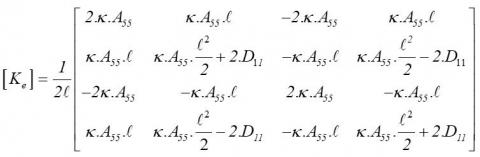(16)

## 17.jpg(17)

The natural frequencies are determined by introducing $v=\phi e^{i \omega x}$ in Eq. (14), gives the problem of quadratic eigenvalue according to:

$\left[\omega^{2} M+\omega C+K\right] \phi=0$ (18)

$\operatorname{det}\left|\omega^{2} M+\omega C+K\right|=0$ (19)

The solution of Eq. (19) produces 2n eigenvalue and 2n corresponding eigenvectors (i = 1, ..., 2n), which represent the modes and the natural frequency of vibratory system. As the problem considered here is unconventional, the eigenfrequencies occur in the conjugate complexes of the form:

$\omega_{1}=-\omega_{N, I} \zeta_{1}+i \omega_{N, I}(\sqrt{1-\zeta_{1}^{2}})=-\omega_{N, 1} \zeta_{1}+i \omega_{d, 1}$ (20)

When the damping matrix [C] equals 0, the solution problem of equation (14) is considered conventional, representing the free vibration behavior of the FGM beam, in the form:

$\operatorname{det}\left[K-\omega^{2} M\right]=0$ (21)

6. Numerical Results and Discussion

The vibration analysis of the FGM beams is carried out by the Timoshenko model with internal viscous damping distribution (DIVD). The numerical application is presented on the cantilever functionally graded beams assuming that the height (h) and width (b) of the beam are uniform (h=b=0.02 m). The FGMs is made of a mixture of ceramic and metal whose composition varies across the thickness direction through the power low function. As the upper facet i.e., at (z = h / 2) is made of 100% ceramic Al2O3 (Alumina), while the lower facet (z = -h / 2) is made in 100 % metal Al (Aluminum). The material properties of Al2O3 are:

Ec=380GPa, ρc=3800Kg/m3, υc=0.23 and those of Al are: Em=70GPa, ρm=2700Kg/m3, υm=0.23

Table 1 presents a comparison of the undamped fundamental frequencies results of the FGM cantilever beam for different values of power law index ‘’p’’ and the aspect ratio (L/h). The dimensionless undamped eigenfrequencies are defined as:

$\bar{\omega}_{n}=\omega_{n} \frac{L^{2}}{h} \sqrt{\frac{\rho_{m}}{E_{m}}}$

The results obtained are compared with the analytical results proposed by (Nguyen et al.) . It can be seen that the numerical results of this model show an excellent agreement for both deep and thin FGM beams. We also note that when the Number of Element “NE” increases the values of the free pulsation converges to reliable values.

Figure 5 shows an analysis of the first damped frequencies for different curves corresponding to four dimension ratio values (L/h), varying the internal damping coefficient β and the volume fraction of the material (p).

The introduction of the internal damping into the vibratory system induces a further variation in the vibratory frequencies of the FGM beam, the increase of damping coefficient β implies a decrease of the damped frequency, and this change becomes very crucial for the beams that have high rigidity and low slenderness (L/h).

Table 1. Comparison of dimensionless undamped frequencies

 L/h NE Full Ceramic p=0.5 p=1 p=5 p=10 Full Metal 05 elements 1.9030 1.6820 1.5966 1.4234 1.3132 0.9888 10 elements 1.8966 1.6763 1.5913 1.4186 1.3088 0.9855 5 50 elements 1.8945 1.6745 1.5895 1.4171 1.3073 0.9844 100 elements 1.8944 1.6744 1.5894 1.4170 1.3073 0.9843 (Nguyen et al.)  1.8957 1.6182 1.4636 1.2594 1.2187 - 05 elements 1.9586 1.7294 1.6433 1.4748 1.3614 1.0177 10 elements 1.9519 1.7235 1.6376 1.4698 1.3567 1.0142 20 50 elements 1.9496 1.7215 1.6357 1.4681 1.3551 1.0130 100 elements 1.9496 1.7214 1.6357 1.4680 1.3551 1.0130 (Nguyen et al.)  1.9496 1.6602 1.5011 1.3034 1.2646 -

## 51.png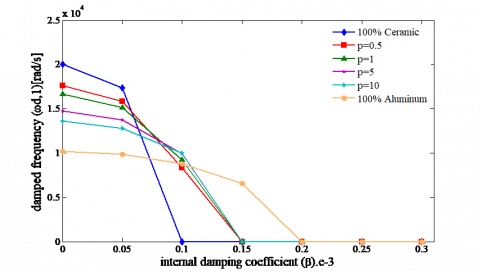a)

## 52.png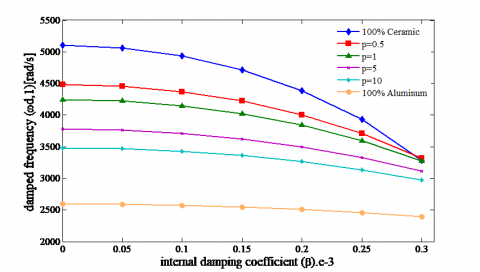b)

## 53.pngc)

## 54.pngd)

Figure 5. Effect of the internal damping coefficient on the damped frequencies for  a) L/h = 5; b) L/h = 10; c) L/h = 15; d) L/h = 20

7. Conclusion

The FGMs structures represent a vast field of research and applications so huge it can change their composition and geometry for optimal properties.

In this paper, we presented theoretical research on damped vibrations functionally graded beams based on a mixture of two different materials, using the Timoshenko beam theory (FSDBT) and DIVD model. The material properties vary through the thickness direction of the beam according to the power law distribution (P-FGM).

Several physical and geometrical parameters are processed to analyze the dynamic stability of FGM Timoshenko beams. The main conclusions of this study are:

• The damped frequencies are inversely proportional to the internal damping coefficient (β).
• The change in the eigenfrequencies depends on the combination of the volume fractions of the reference materials.
• The slenderness ratio (L/h) has a noticeable effect on the variation of the damped fundamental frequencies.
• The effectiveness of the distributed internal viscous damping (DIVD) can be manipulated according to the choice of the material properties in the FGM beams.
Nomenclature
 L Total Length of beam, m h Thickness of the beam, m b Width of beam, m $\ell$ Elementary length of the beam p Power index E Young's module, Gpa $\left[M_{e}\right]$ Elementary mass matrix $\left[C_{e}\right]$ Elementary damping matrix $\left[K_{e}\right]$ Elementary matrix of stiffness $I_{1}, I_{2}$ Function of the volume fraction $\mathcal{R}$ Rayleigh dissipation function T Kinetic energy V Potential Energy $[Q]$ The Hookean elasticity tensor Greek symbols $\left\{\sigma^{T}\right\}$ Total stress tensors $\left\{\sigma^{C}\right\}$ Bending and shear classical stress $\left\{\sigma^{\mathrm{d}}\right\}$ Bending and shear dissipative additional stress $\{\varepsilon\}$ The strain tensor $\{\dot{\varepsilon}\}$ The strain rate tensor $\beta$ Global viscous internal damping coefficient $\rho$ Mass density, Kg.m-3 $\boldsymbol{K}$ The shear correction factor of the Timoshenko beam theory u Displacement along the X axis Ɵ Rotation along the X axis ν Poisson coefficient $\omega$ Eignfrequency of the FGM beam $\omega_{N, 1}$ Unamortized natural frequency $\zeta_{1}$ The modal damping factor $\omega_{d, 1}$ The damped natural frequency $\mathscr{Q}$ Lagrangian fonction
References

 Sahoo, A.K. (2014). Static, free vibration and dynamic stabilty analysis of sandwich beam with functionally graded material constraining layer in high temperature environment (Doctoral dissertation). National Institute of Technology Rourkela-769008, India.

 Berthier, P. (1973). Étude dynamique de certains types de structures: Amortissement par revêtement viscoélastique (Doctoral dissertation). university, country.

 Gjika, K. (1994). Comportement dynamique de structures localement non lineaire: Cas des suspensions (Doctoral dissertation, Lyon, INSA). university, country.

 Nashif, A.D., Jones, D.I., Henderson, J.P. (1985). Vibration Damping. John Wiley & Sons.

 Kaskas, S. (2010). Etude de l'effet du cisaillement transverse sur le comportement mécanique de poutres composites. Thèse de Magister en génie civil, Université Mentouri de Constantine, Algérie.

 Gorman, D.J. (1975). Free Vibration Analysis of Beams and Shafts (Book). Research supported by the National Research Council of Canada. New York, Wiley-Interscience, p. 395.

 Hassan, A.H.A., Kurgan, N. (2019). A Review on buckling analysis of functionally graded plates under thermo-mechanical loads.  International Journal of Engineering & Applied Sciences, 11(1): 345-368. http://dx.doi.org/10.24107/ijeas.555719

 Duc, N.D., Tung, H.V. (2010). Mechanical and thermal post buckling of shear-deformable FGM plates with temperature-dependent properties. Mechanics of Composite Materials, 46(5): 461-476. https://doi.org/10.1007/s11029-010-9163-9

 Reddy, J.N., Cheng, Z.Q. (2001). Three-dimensional thermomechanical deformations of functionally graded rectangular plates. European Journal of Mechanics-A/Solids, 20(5): 841-855. https://doi.org/10.1016/S0997-7538(01)01174-3

 Fallah, F., Nosier, A., Sharifi, M., Ghezelbash, F. (2016). On perturbation method in mechanical, thermal and thermo-mechanical loadings of plates: Cylindrical bending of FG plates. ZAMM-Journal of Applied Mathematics and Mechanics/Zeitschrift für Angewandte Mathematik und Mechanik, 96(2): 217-232. http://dx.doi.org/10.1002/zamm.201400136

 Elmeiche, A., Megueni, A., Lousdad, A. (2016). Free vibration analysis of functionally graded Nanobeams based on different order beam theories using Ritz method. Periodica Polytechnica Mechanical Engineering, 60(4): 209-219. http://dx.doi.org/10.3311/PPme.8707

 Aydogdu, M., Taskin, V. (2007). Free vibration analysis of functionally graded beams with simply supported edges. Materials & Design, 28(5): 1651-1656. http://dx.doi.org/10.1016/j.matdes.2006.02.007

 Bouamama, M., Refassi, K., Elmeiche, A., Megueni, A. (2018). Dynamic behavior of sandwich FGM beams. Mechanics and Mechanical Engineering, 22(4): 919-929.

 Alshorbagy, A.E., Eltaher, M.A., Mahmoud, F.F. (2011). Free vibration characteristics of a functionally graded beam by finite element method. Applied Mathematical Modelling, 35(1): 412-425. http://dx.doi.org/10.1016/j.apm.2010.07.006

 Eltaher, M.A., Emam, S.A., Mahmoud, F.F. (2013). Static and stability analysis of nonlocal functionally graded nanobeams. Composite Structures, 96: 82-88. http://dx.doi.org/10.1016/j.compstruct.2012.09.030

 Ebrahimi, F., Barati, M.R. (2017). Hygrothermal effects on vibration characteristics of viscoelastic FG nanobeams based on nonlocal strain gradient theory. Composite Structures, 159: 433-444. http://dx.doi.org/10.1016/j.compstruct.2016.09.092

 Elmeiche, A., Bouamama, M., Megueni, A. (2018). Dynamic analysis of FGM nanobeams under moving load considering shear deformation effect. International Journal of Scientific & Engineering Research, 9(3): 1212-1221.

 Rahmani, O., Pedram, O. (2014). Analysis and modeling the size effect on vibration of functionally graded nanobeams based on nonlocal Timoshenko beam theory. International Journal of Engineering Science, 77: 55-70. http://dx.doi.org/10.1016/j.ijengsci.2013.12.003

 Sorrentino, S., Marchesiello, S., Piombo, B.A.D. (2003). A new analytical technique for vibration analysis of non-proportionally damped beams. Journal of Sound and Vibration, 265(4): 765-782. http://dx.doi.org/10.1016/S0022-460X(02)01560-2

 Dohnal, F., Ecker, H., Springer, H. (2008). Enhanced damping of a cantilever beam by axial parametric excitation. Archive of Applied Mechanics, 78(12): 935-947. http://dx.doi.org/10.1007/s00419-008-0202-0

 Robu, B., Baudouin, L., Prieur, C. (2011). Contrôle actif des vibrations dans un système fluide/structure. Journal Européen des Systèmes Automatisés (JESA), 45(7): 495-511. http://doi.org/10.3166/jesa.45.495-511

 Chang, T.P., Chang, F.I., Liu, M.F. (2001). On the eigenvalues of a viscously damped simple beam carrying point masses and springs. Journal of Sound Vibration, 240: 769-778. https://doi.org/10.1006/jsvi.2000.3186

 Lin, S.M., Lee, J.F., Lee, S.Y., Wang, W.R. (2006). Prediction of vibration of rotating damped beams with arbitrary pretwist. International journal of mechanical sciences, 48(12): 1494-1504. https://doi.org/10.1016/j.ijmecsci.2006.05.015

 Chen, W.R. (2011). Bending vibration of axially loaded Timoshenko beams with locally distributed Kelvin–Voigt damping. Journal of Sound and Vibration, 330(13): 3040-3056. https://doi.org/10.1016/j.jsv.2011.01.015

 Bendine, K., Satla, Z., Boukhoulda, F.B., Nouari, M. (2018). Active Vibration damping of Smart composite beams based on system identification technique. Curved and Layered Structures, 5(1): 43-48. http://dx.doi.org/10.1515/cls-2018-0004

 Capsoni, A., Viganò, G.M., Bani-Hani, K. (2013). On damping effects in Timoshenko beams. International Journal of Mechanical Sciences, 73: 27-39. http://dx.doi.org/10.1016/j.ijmecsci.2013.04.001

 Van de Vegte, J., de Silva, C.W. (1976). Design of passive vibration controls for internally damped beams by modal control techniques. Journal of Sound and Vibration, 45(3): 417-425. https://doi.org/10.1016/0022-460X(76)90396-5

 Tsai, T.C., Tsau, J.H., Chen, C.S. (2009). Vibration analysis of a beam with partially distributed internal viscous damping. International Journal of Mechanical Sciences, 51(11-12): 907-914. https://doi.org/10.1016/j.ijmecsci.2009.09.039

 Chi, S.H., Chung, Y.L. (2006). Mechanical behavior of functionally graded material plates under transverse load—Part I: Analysis. International Journal of Solids and Structures, 43(13): 3657-3674. https://doi.org/10.1016/j.ijsolstr.2005.04.011

 Bao, G., Wang, L. (1995). Multiple cracking in functionally graded ceramic/metal coatings. International Journal of Solids and Structures, 32(19): 2853-2871. https://doi.org/10.1016/0020-7683(94)00267-Z

    Nguyen, T.K., Nguyen, T.T.P., Vo, T.P., Thai, H.T. (2015). Vibration and buckling analysis of functionally graded sandwich beams by a new higher-order shear deformation theory. Composites Part B: Engineering, 76: 273-285. https://doi.org/10.1016/j.compositesb.2015.02.032